#### Last visited songs

No songs visited yet...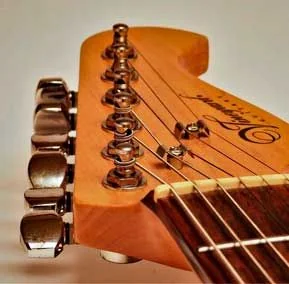# Canned Music chords by Dan Hicks## Chords with lyrics

```Canned Music    Dan Hicks                  NOTES BELOW
[G7G7]
(C majorC) Canned ..
[C majorC ]                             [G7G7]
Canned ....
[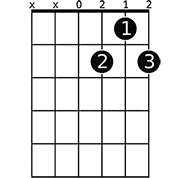D7D7]                         [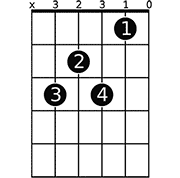C7C7]
Favorites ...
[G7G7]
Canned ..

[G7G7]
(1)A little ..
[C7C7]                  [C majorC ] [C7C7] [G7G7]
She ..
[D7D7]                               [C7C7]
I took ..
[G7G7]
Live ...

(2)She got ..
I ..=

I ..
Live ..

* Repeat (C majorC)
* Instrumental

(3)The ..
My ..
ut
She ..
It's ..
(4)And ..
My ..
that mystery
I think ..
it's
Live ..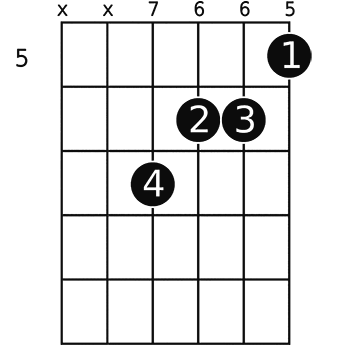A augmentedA ..
She ...
.....................................................................=

Some chord idea's.

X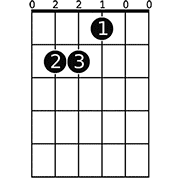E MajorEA augmentedA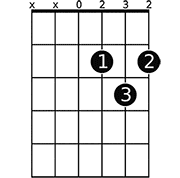D MajorD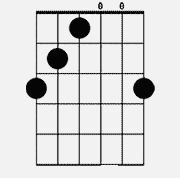G+G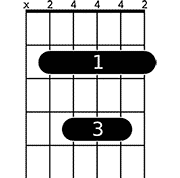BBE MajorE
C7...=D5=CD=D1=CD=D1=CD=D1=CD=D1=CD=B8C7C7 =D5=CD=D1=CD=D1=CD=D1=CD=D1=
=CD=B8        D7=3D THIS FIGURE MOVED UP TO
=B3 =B3 =B3 =B3 =B3 =B3     =B3 =B3 =B3 =B3 =B3 =B3  <-------- BA=
RRE 5th FRET
up 2  =C3=C4=C5=C4=C5=C4=C5=C4=C5=C4=B4     =C3=C4=C5=C4=C5=C4=C5=C4=C5=
=C4=B4
frets =B3 =B3 1 =B3 =B3 =B3     =B3 =B3 =B3 =B3 =B3 =B3
for   =C3=C4=C5=C4=C5=C4=C5=C4=C5=C4=B4     =C3=C4=C5=C4=C5=C4=C5=C4=C5=
=C4=B4D7D7    =B3 2 =B3 3 3 3     1 1 1 1 1 1 BARRE at 3rd
=B3 =B3 =B3 =B3 =B3 =B3     =C3=C4=C5=C4=C5=C4=C5=C4=C5=C4=B4
o o       =B3 =B3 =B3 =B3 =B3 =B3
=D5=CD=D1=CD=D1=CD=D1=CD=D1=CD=B8     =C3=C4=C5=C4=C5=C4=C5=C4=C5=
=C4=B4
G7=3D =B3 =B3 =B3 =B3 =B3 1     =B3 =B3 3 =B3 4 =B3 <---Also try movi=
ng 4th finger toE MajorE string
=C3=C4=C5=C4=C5=C4=C5=C4=C5=C4=B4     =C3=C4=C5=C4=C5=C4=C5=C4=C5=
=C4=B4     I don't know what it's called.
=B3 2 =B3 =B3 =B3 =B3     =B3 =B3 =B3 =B3 =B3 =B3     I call it h=
ere..C?
=C3=C4=C5=C4=C5=C4=C5=C4=C5=C4=B4
3 =B3 4 =B3 =B3 =B3     This is kind of rough. On the CD they do =
this in G,
=B3 =B3 =B3 =B3 =B3 =B3     but I'm going to fool around with oth=
er keys as well,
to try and find that sound that's so elusive.
What'cha think ? I think it could be improved, ju=
st
don't know how.
[email protected]
[email protected]       RIME->203
=1A=

--PART.BOUNDARY.0.396.emout04.mail.aol.com.810447725--```

### Welcome to the guitar community

- Get songbook with favourite songs
- Get custom made front page
- Submit chords and tabs
- Submit guitar-cover videos
- Get a lot of thumbs up for your work

#### Last visited songs

No songs visited yet...

Site
Home
Search
Community
Submit chords
Submit video

### Video

No video for this page. Check out how you can submit guitar-cover videos here